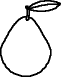EnchantedLearning.com is a user-supported site.
As a bonus, site members have access to a banner-ad-free version of the site, with print-friendly pages.

 You might also like: Divide Fish by Five Worksheet Printout Divide Fruit into Three Groups Worksheet Printout Divide Fruit into Four Groups Worksheet Printout Divide Eight Birds Worksheet Printout Visual Division #3 Worksheet Printout Today's featured page: Printing Tips

 Go to the AnswersMath Pages EnchantedLearning.com Divide Fruit into Two Groups Divide the fruit into groups to do division. Visual Division PagesDivision Pages

 Divide the pears into two equal groups.How many pears are there? How many groups of pears are there? How many pears are in each group?
4 ÷ 2 =

 Divide the bananas into two equal groups.How many bananas are there? How many groups of bananas are there? How many bananas are in each group?
6 ÷ 2 =

 Divide the lemons into two equal groups.How many lemons are there? How many groups of lemons are there? How many lemons are in each group?
8 ÷ 2 =

## Enchanted Learning Search

 Search the Enchanted Learning website for: